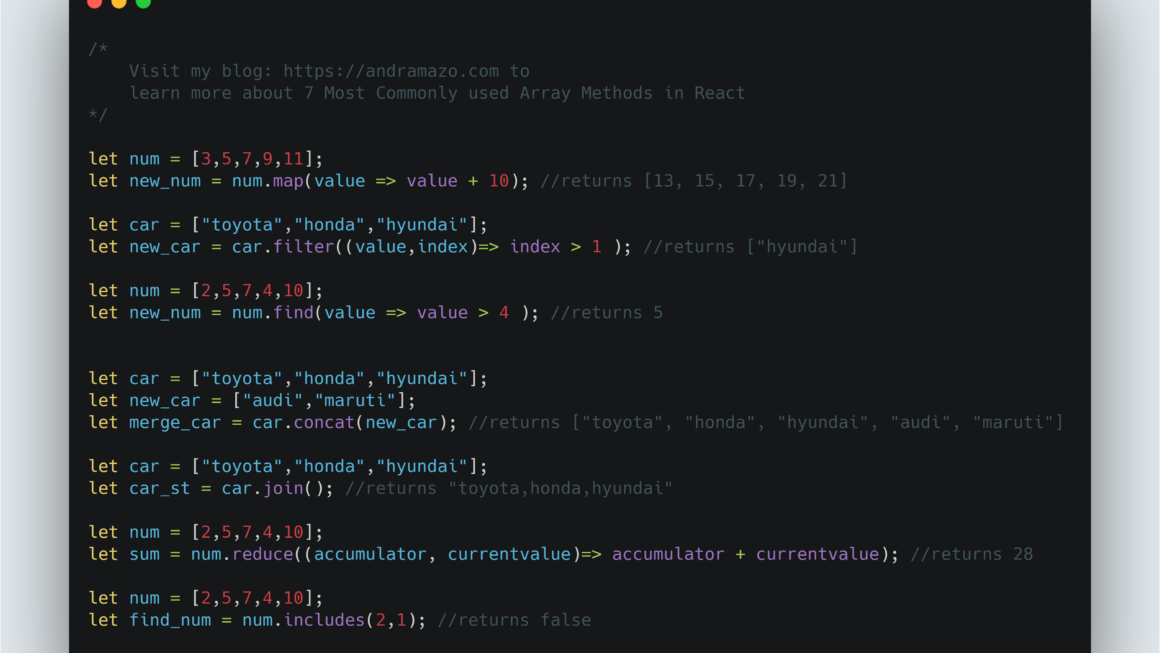# 7 Most Commonly used Array Methods in ReactReact is the most popular front end library. Knowing most commonly used array methods makes the development process easier.

map() -function returns the new array where it takes a function that will be triggered on each element of the provided array. function takes first argument as each element of an given array. The second argument is optional but it provides the index of the given element.

``````let num = [3,5,7,9,11];
let new_num = num.map(value => value + 10); //returns [13, 15, 17, 19, 21]

let car = ["toyota","honda","hyundai"];
let new_car = car.map((value,index)=> value + " at " + index); // returns ["toyota at 0", "honda at 1", "hyundai at 2"]``````

filter() – Returns the new array with all the element passed for the provided filter for the array. Just like map method, this method function has second optional parameter which is the index of the current element.

``````let num = [2,5,7,4,10];
let new_num = num.filter(value => value % 2); //returns [5, 7]

let car = ["toyota","honda","hyundai"];
let new_car = car.filter((value,index)=> index > 1 ); //returns ["hyundai"]``````

find() – Returns the first element which satisfies the provided filter. This method function has second optional parameter which is the index of the current element.

``````let num = [2,5,7,4,10];
let new_num = num.find(value => value > 4 ); //returns 5``````

reduce() – Returns a single value for the provided array on each element. Function takes two required parameters – accumulator and current value and two optional parameter – current index and source array.

``````let num = [2,5,7,4,10];
let sum = num.reduce((accumulator, currentvalue)=> accumulator + currentvalue); //returns 28``````

join() – Returns a new string by concatenating all the elements of the provided array. The function accepts the optional parameter which is used for separating the string. If it is not provided it will separate the element by ‘,’. If empty string is provided as parameter, it will not put any separator.

``````let car = ["toyota","honda","hyundai"];
let car_st = car.join(); //returns "toyota,honda,hyundai"
let car_new = car.join('_'); //returns "toyota_honda_hyundai"
let car_new1 = car.join(''); //returns "toyotahondahyundai"``````

concat() – Returns a new array by joining two or more arrays.

``````let car = ["toyota","honda","hyundai"];
let new_car = ["audi","maruti"];
let merge_car = car.concat(new_car); //returns ["toyota", "honda", "hyundai", "audi", "maruti"]``````

includes() – Returns a boolean values depending on whether the array includes the expected value provided as parameter to the function. It also accepts the optional parameter where you can define from which index, the search should begin. In the example below, you can see when the index is provided as the second parameter, it starts searching “2” from index 1. Even though 2 is there in the array, it will return false.

``````let new_car = ["audi","maruti"];
let is_toyota = new_car.includes('toyota'); //returns false
let is_audi = new_car.includes('audi'); //returns true

let num = [2,5,7,4,10];
let find_num = num.includes(2,1); //returns false``````